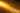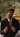Speed of Light, Theory of Special Relativity

Why is the speed of light a finite constant?Govindswaroop Rahangdale

Sep 03, 2020

Why does information travel with a finite and constant speed? In simple words, why is it that always the cause of an event precedes the effect of the event?

As Einstein did it, let’s conduct a thought experiment. Suppose I have two balls, both contain the information regarding an event. Ball 1 carries the information regarding the cause of an event, and Ball 2 carries the information regarding the effect of the event. I throw Ball 1 at you, standing some distance away from me, with a specific but very slow speed. A moment later, I throw Ball 2 at you with a much much higher speed. What happens?

You receive Ball 2 earlier than Ball 1. This means you received the information regarding the effect of the event earlier than the information regarding the cause of the event. In physical reality, this result signifies that a dog died without being born, or a bullet hits a bird without being fired, and so on, which is absurd. Thus, from this thought experiment, we infer that any information should travel at a constant and finite speed, i.e., the light should travel at a constant speed too.

James Clerk Maxwell, in 1861-62, introduced the famous Maxwell’s equations using which, in 1864, he calculated the speed of light in a vacuum, as the ratio of magnetic field component amplitude and electric field component amplitude, to be given by:

c = 1 / (ε0μ0)^ 1/2

Where ε0 is the permittivity of vacuum, and μ0 is the permeability of free space. Afterward, when the speed of light was calculated to be c = 2.998 x 108 m/s, it was too close to the value calculated by Maxwell in 1864.

The speed of information travel being constant is a very general result in Einsteins’s theory of Special Relativity.